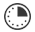# Order of Operations

Brittany Zae
Teacher

This lesson is designed to help students solve problems involving the four operations, and identify and explain patterns in arithmetic.

##Length of Time: About 45 Minutes

Featured Programs:

### Common Core Alignment

CCSS: MATH.CONTENT.4.OA.A.3 - Solve multistep word problems posed with whole numbers and having whole-number answers using the four operations, including problems in which remainders must be interpreted. Represent these problems using equations with a letter standing for the unknown quantity. Assess the reasonableness of answers using mental computation and estimation strategies including rounding.

## Objectives & Outcomes

Students will be able to apply order of operations when computing with whole numbers, excluding exponents.

## Materials Needed

• popsicle sticks
• peanut butter
• jelly
• knife
• napkin

## Procedure

### Opening to Lesson

• Ask students how to make a peanut butter and jelly sandwich. Have students write down their directions with a partner.
• CFU: As the students give their directions, make the sandwich as a demonstration for the class, doing EXACTLY what the students say.

### Body of Lesson

#### Direct Teaching

• Talk with students about the importance of giving exact directions in the right order, not only when making a peanut butter and jelly sandwich, but when choosing the order of operations when solving a problem. Just like with sandwiches, if we go out of order, we won’t get the correct result… review order of operation on board.
• CFU: Discuss introduction activity and go over the order of operation and corresponding rules *Remind students that the multiplication and division steps are a group which work from left to right: multiplication does not come before division. This is the same with addition and subtraction.

#### Guided Practice 1

• Show students a sample math problem: “To find out the answer to this problem you must use the order of operations, which means that all of the operations you have already learned must be calculated in a certain order: PEMDAS (Parentheses, Exponents, Multiplication & Division, Addition & Subtraction)."
• CFU: Solve one problem as a class. Give the stick example 7- (2+1) and show how the answer is 4. Explain that if you ignore the parentheses and just go in order from left to right, you get 6 as your answer. But if you start inside the parentheses (adding 2 and 1 to get 3, you get the correct answer of 4.

#### Guided Practice 2

• Complete the 2 problems on their worksheet and compare answers with their partner.
• Example 1: 4+5 x 6 -7 | Mult. 5 x 6=30 | Add. 4 + 30-7=34-7 | Sub. 34-7=27 |
• Example 2: (Real World): Joe buys 2 shirts at \$8.00 each. He also buys a pair of jeans for \$20.00 that gets a \$3.00 discount. Write a numerical expression and solve. (2 x \$8.00)+(\$20.00-\$3.00)
|Par. (2 x 8)=16 and (20 - 3)=17 | Addition \$16+\$17=\$33
• Example 3: 4 x ((3 x (2^2)-1)
| Par ((3 x (2^2)-1) | Exp. 2^2=4 |Mult. 3 x 4 -1 | Sub. 12-1=11

#### Independent Practice

• Ask students to work as a group to put their dominoes in the correct square. Have students connect the answers (one side of the stick) and equations (second side of the stick) to form a domino chain.
• CFU: Observe as they work together in groups.

### Closing

• Choose on student groups as they read aloud their equations.  Have students check to see if they are correct.
• Option: Swap seats and have them review to see if they are correct… or check to see where they can break apart their square and connect to the other group.

## Assessment & Evaluation

Through guided questions, close monitoring and informal observation; the teacher will be able to assess student’s ability and understanding of the subject being introduced. Through individually assessing during independent practice and reviewing during guided practice the teacher will be able to evaluate students.

## Related Lesson Plans

##### Symmetry Search

The students will locate manmade objects or things in nature that are symmetrical.

##### Numbers By The Book

This lesson will allow students to show a relationship between numbers and a visual representation in a manner that can be used for younger students.

##### Comparing Decimals Game

Students will play a game in which they choose cards and choose the best place to put the number they have chosen in order to get the highest answer possible.  This should not be the first introduction to the topic.

##### Fractions, Decimals, Percents, & Graphs

This lesson is designed to explore fractions, decimals, percent’s, and graphs with TRIX cereal.